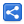# Java Collections - How to find frequency of each element in a collection?

[Last Updated: Apr 28, 2020]

Following example shows how to find how many times each element is repeated (i.e. frequency of each element) in a collection.

# Example

## Frequency of a particular element

To find frequency of a particular element we can use `java.util.Collections#frequency()` method:

```package com.logicbig.example;

import java.util.Arrays;
import java.util.Collections;
import java.util.List;

public class CollectionUtil {
public static void main(String[] args) {
List<Integer> list = Arrays.asList(1, 3, 4, 5, 3, 2, 2, 4, 2);
int frequency = Collections.frequency(list, 2);
System.out.println(frequency);
}
}
```
`3`

## Frequencies of all elements

Following example shows how to find frequency of each element in a collection:

```package com.logicbig.example;

import java.util.*;

public class CollectionUtil2 {
public static void main(String[] args) {
List<Integer> list = Arrays.asList(1, 3, 4, 5, 3, 2, 2, 4, 2);
Map<Integer, Integer> frequencyMap = new HashMap<>();
list.stream()
.distinct()
.forEach(element -> frequencyMap.put(element, Collections.frequency(list, element)));
System.out.println(frequencyMap);
}
}
```
`{1=1, 2=3, 3=2, 4=2, 5=1}`

 Share